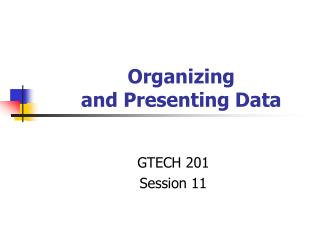Download PresentationOrganizing and Presenting Data

# Organizing and Presenting Data - PowerPoint PPT Presentation

Organizing and Presenting Data. GTECH 201 Session 11. Terminology. Classes Categories for grouping data Frequency Number of observations that fall in a class (frequency is a count) Frequency Distribution A listing of all classes along with their frequencies Relative FrequencyI am the owner, or an agent authorized to act on behalf of the owner, of the copyrighted work described.
Download Presentation## Organizing and Presenting Data

An Image/Link below is provided (as is) to download presentation

Download Policy: Content on the Website is provided to you AS IS for your information and personal use and may not be sold / licensed / shared on other websites without getting consent from its author.While downloading, if for some reason you are not able to download a presentation, the publisher may have deleted the file from their server.

- - - - - - - - - - - - - - - - - - - - - - - - - - E N D - - - - - - - - - - - - - - - - - - - - - - - - - -
Presentation Transcript
1. Organizing and Presenting Data GTECH 201 Session 11

2. Terminology • Classes • Categories for grouping data • Frequency • Number of observations that fall in a class (frequency is a count) • Frequency Distribution • A listing of all classes along with their frequencies • Relative Frequency • The ratio of the frequency of a class to the total number of observations • Relative Frequency Distribution • A listing of all classes along with their relative frequencies • Width/Class Interval • The difference between the upper and lower cut points (breaks) of a class

3. Organizing Data • Classification Rules • Aim is to create categories or classes • First step is to compute range • Range = Largest Value – Smallest Value • Interval or Ratio Scale data only • Class Intervals • Width of Class Interval • Equal based on range • Unequal based on range • Quantile (Quartile or Quintile) • Natural

4. Classification Methods • Natural breaks • Quantile • Manual • Equal interval

5. How to Decide(on a classification scheme) • Rule of thumb: 3 - 7 classes • Classification histogram (see later today)

6. How to Decide, part II

7. Graphs • Line graph • Bar graph • Scatterplots

8. Creating a Line Graph • The growth of the population of students at a Midwestern university is as follows

9. Line Graph

10. Bar Graphs • Here are data on the percent of females among people earning doctoral degrees in 1990, in several different fields of study

11. Bar Graph

12. Scatter Plots • Graph bi-variate data when both variables are measured in an interval/ratio or ordinal scale • Units for one variable are marked on the horizontal axis • Independent variable should always go on the horizontal, x axis

13. Scatterplots • Survey of 3368 people asking them to estimate number of calories in common foods.

14. Example • A city planner collected data on the number of school age children in each of 30 families. • Construct a grouped data table using classes based on a single value

15. Computing Frequency • There are three ways you can create classes • a < but not equal to b b < but not equal to c • a – b, c – d, e - f • single value grouping

16. Distributions • Histograms • Difference between histograms and bar graphs • Bars in a histogram are always vertical • Base scale is marked off in equal units; there is no base scale in a bar graph • Width of bars in a histogram have meaning • Bars in a histogram touch each other

17. Constructing a Histogram • Histogram – height of bar equal to frequency of class represented • Bar extends from lowest value to highest value of the class

18. Histogram Chart

19. Frequency Polygons • Similar to a histogram • Midpoint of the class is indicated • Points connected by straight lines • Cumulative frequency polygon, ogive